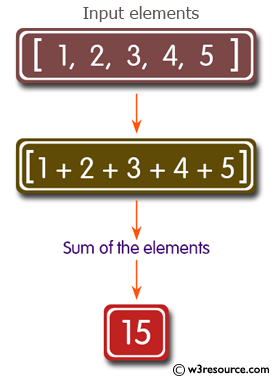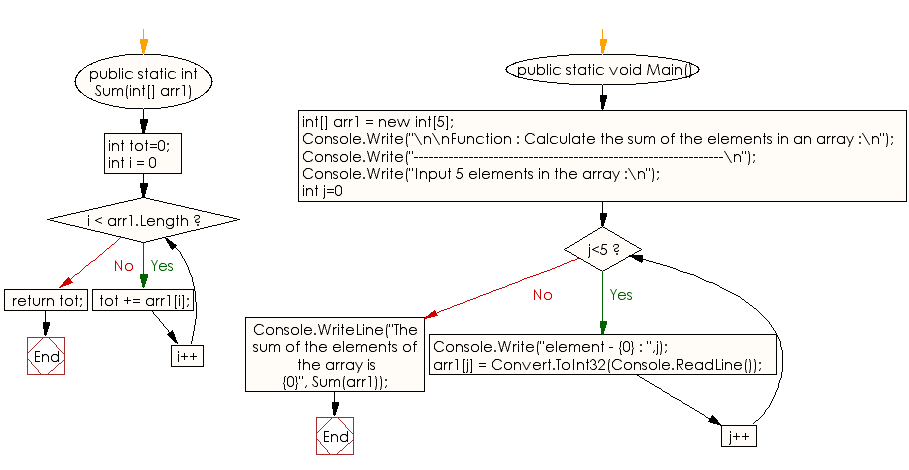﻿ C# Sharp Exercises: Function : Calculate the sum of the elements in an array - w3resource# C# Sharp Exercises: Function: Calculate the sum of the elements in an array

## C# Sharp Function: Exercise-5 with Solution

Write a program in C# Sharp to calculate the sum of elements in an array.

Pictorial Presentation:Sample Solution:

C# Sharp Code:

``````using System;
public class funcexer5
{
public static int Sum(int[] arr1)
{
int tot=0;
for (int i = 0;i < arr1.Length; i++)
tot += arr1[i];
return tot;
}
public static void Main()
{
int[] arr1 = new int;
Console.Write("\n\nFunction : Calculate the sum of the elements in an array :\n");
Console.Write("--------------------------------------------------------------\n");

Console.Write("Input 5 elements in the array :\n");
for(int j=0; j<5; j++)
{
Console.Write("element - {0} : ",j);
arr1[j] = Convert.ToInt32(Console.ReadLine());
}
Console.WriteLine("The sum of the elements of the array is {0}", Sum(arr1));
}
}
```
```

Sample Output:

```Function : Calculate the sum of the elements in an array :
--------------------------------------------------------------
Input 5 elements in the array :
element - 0 : 1
element - 1 : 2
element - 2 : 3
element - 3 : 4
element - 4 : 5
The sum of the elements of the array is 15
```

Flowchart:C# Sharp Code Editor:

Improve this sample solution and post your code through Disqus

What is the difficulty level of this exercise?

﻿